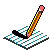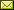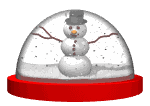PHYSICS@HORTONPhysics12

Physics 11

Math 11

NS Exams

Curriculum

Tips

Tutorials

McGraw Hill

Resources

Need it?

News

Enrichment

GiancoliIB Physics SL

April 2019 Notes

 March 29 & April 1 Projectile Motion Penny Demo Projectile launched and dropped at same time Shoot-n-Drop Video Analysis of Projectile Motion (Ball Toss ~ Physics Tool Kit) Video Analysis of Projectile Motion (Bird Toss in moving chair ~ Logger Pro) Notes Projectile Practice from McGraw-Hill (see post in Google Classroom) Notes work on LABS, Popper Physics, Reaction Time work on 2-1 TO DO List April 2 Error Analysis Lab Motion on an Incline (not in 2019) Investigating the Fall of a Coffee Filter (Design) (not in 2019) April 3 KINDNESS DAY April 4 PRESCRIBED PRACTICAL ~ Measuring the acceleration due to gravity by timing a freefalling object (write up as DCP/CE) Try the following simulation: Free Fall Model April 5 QUIZ (2.1 Kinematics) work on Labs April 8 Forces (Weight/Normal Force/FBDs) BrainPop: Gravity What is Gravity? What is a Force? What Forces are Acting on You? Forces and Motion Simulation Lab Notes April 9STORM DAY Work at home: Forces (Resultant Forces/Balanced & Unbalanced Forces/Newton's First Law/Translational Equilibrium/Newton's Second Law) Notes April 10 Trig or Treat (for October!) Forces in 1D (needs Java) Finish "Popper Physics" Work on "TO DO" list April 10 April 11 Forces (Newton's Second Law) BrainPop: Force Forces & Motion: Basics (Tug of War & Acceleration Lab) (needs Java) Negative Mass?? Notes Forces (Friction) Forces (Fluid Resistance) The Physics Classroom The Calculator Pad Notes April 12 Investigating the Terminal Velocity of a Coffee Filter April 15 Finish Notes from April 11 Work on Terminal Velocity Lab April 16 Momentum & Impulse (Momentum, Impulse & Newton's Second Law/F-t graphs/Conservation of Linear Momentum) Momentum & Impulse More Momentum & Impulse Impulse Bouncing Ball Momentum & Collisions (PHYSCLIPS) What is wrong in the NS Driver's Handbook? (see p51) Notes April 17 Momentum & Impulse (Conservation of Linear Momentum) pHET Collision Lab (needs JAVA!!! ~ settings: 1D, check velocity vectors & momenta diagram, try 100%, 50%, 0% elasticity, try different mass combinations, try different velocities and look at the total mometum before & after collision) 2D Collisions (World-in-Motion video analysis) April 18 Momentum & Impulse (Conservation of Linear Momentum) Cannon Ball Fired from Back of Moving Truck Recoil Velocity Animation Notes1 Notes2 Momentum & Impulse (Conservation of Linear Momentum) Notes Video: Momentum (Vector Addition) ~ see Google Classroom April 23 Momentum & Impulse (Newton's Third Law) Notes April 24 Impulse & Momentum Lab April 25 Work, power, and energy (Forms of energy, conservation of energy, work) BrainPop: Work BrainPop: Inclined Plane Absorb Physics: Types of Energy Notes April 26 Work, power, and energy (Work, force displacement graphs, Hooke's Law) Absorb Physics: Doing Work Hooke's Law Animation Hooke's Law Spring Virtual Hooke's Law Lab Hooke's Law (showing elastic limit) PhET: Hooke's Law Find Ronald's Mass Notes April 29 Work, power, and energy (Kinetic energy, gravitational potential energy, energy transformations) Video Analysis of a Falling Object Video analysis of a Falling Object (with air resistance) BrainPop: Kinetic Energy BrainPop: Potential Energy Notes April 30 QUIZ (forces & impulse/momentum) Work, power, and energy (Elastic Collisions) Energy Conservation in a Pendulum Energy Conservation in a Roller Coaster PhET: Energy Skate Park Notes

{subdirectory}